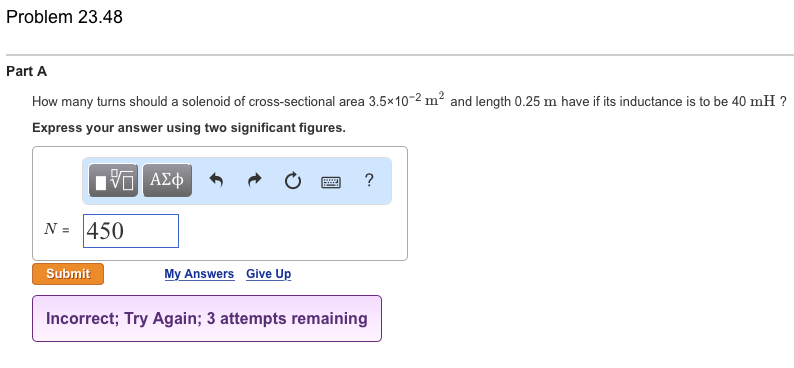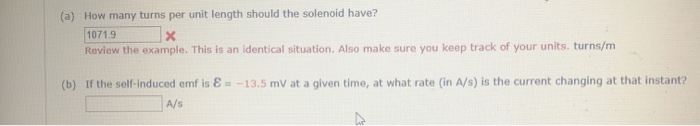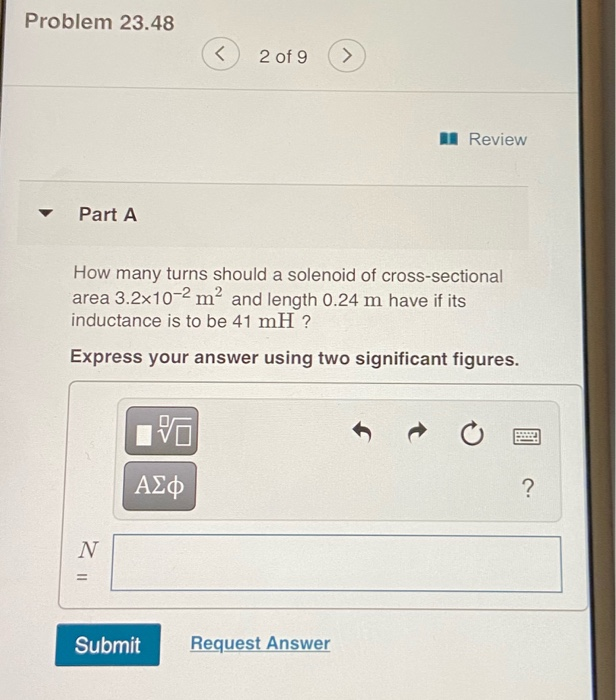Question

How many turns are required to make a solenoid of length .150m and cross sectional area .00325m^2 with a self inducatance of 12.5 mH?

self inducatnce L= mu_o*N^2*A*l

given that A = 0.00325 m^2

l = 0.150 m

mu_o = 4*pi*10^-7 H/m

No.of turns is N = sqrt( L/(mu_o*A*l)= sqrt((12.5*10^-3)/(4*3.142*10^-7*0.00325*0.15)) = 4517 turns

#### Earn Coins

Coins can be redeemed for fabulous gifts.

Similar Homework Help Questions
• ### How many turns should a solenoid of cross-sectional area 3.5times10^-2 m^2 and length 0.25 m have...How many turns should a solenoid of cross-sectional area 3.5times10^-2 m^2 and length 0.25 m have if its inductance is to be 40 mH ? Express your answer using two significant figures.

• ### How many turns should a solenoid of cross-sectional area 4.1×10−2 m2 and length 0.34 m have...

How many turns should a solenoid of cross-sectional area 4.1×10−2 m2 and length 0.34 m have if its inductance is to be 65 mH ? Express your answer using two significant figures.

• ### please help. (a) How many turns per unit length should the solenoid have? 1071.9 x Review...please help. (a) How many turns per unit length should the solenoid have? 1071.9 x Review the example. This is an identical situation. Also make sure you keep track of your units. turns/m (b) If the self-induced emf is € = -13.5 mV at a given time, at what rate (in A/s) is the current changing at that instant? A/s A solenoid is to have an inductance of 0.270 mh, a cross sectional area of A = 5.50 x 10...

• ### The inductance of a solenoid 10.0cm long with a cross-sectional area of 1,00 x 104 m2 is 0.400 mH. How many turns of wire does this solenoid have?

Question 6 The inductance of a solenoid 10.0cm long with a cross-sectional area of 1,00 x 104 m2 is 0.400 mH. How many turns of wire does this solenoid have? 318,000  159,000  564  282 Question 12 A 4 Ohms resistor is connected in parallel with a 20 resistor? 2.0A  2/3 A  3.0A  4/3 A A solenoid with a certain number of turns and carrying a current of 2A has a length of 34cm. If the magnitude of the magnetic field generated at the center of the...

• ### Suppose you wish to make a solenoid whose self-inductance is 1.2 mH. The inductor is to...

Suppose you wish to make a solenoid whose self-inductance is 1.2 mH. The inductor is to have a cross-sectional area of 2.00 10-3 m2 and a length of 0.053 m. How many turns of wire are needed?

• ### Suppose you wish to make a solenoid whose self-inductance is 1.4 mH. The inductor is to...

Suppose you wish to make a solenoid whose self-inductance is 1.4 mH. The inductor is to have a cross-sectional area of 1.7 x 10-3 m2 and a length of 0.059 m. How many turns of wire are needed?

• ### Find the cross-sectional area of a 5.4 cm long solenoid with 520 turns and a self-inductance...

Find the cross-sectional area of a 5.4 cm long solenoid with 520 turns and a self-inductance of 43 µH.

• ### Find the cross-sectional area of a 5.0 cm long solenoid with 500 turns and a self-inductance...

Find the cross-sectional area of a 5.0 cm long solenoid with 500 turns and a self-inductance of 40 pJ-I. Please explain the process to get the answer.

• ### The solenoid shown below has length l = 0.15 m and cross-sectional area A = 3.5...

The solenoid shown below has length l = 0.15 m and cross-sectional area A = 3.5 × 10-4 m2. Its self-inductance is L = 25 mH. A current I of 2.5 amps flows through its coils in the direction shown in the figure. 2. How much magnetic energy, Um, is stored in this solenoid? 3.What is the magnetic field inside the solenoid. (a)   B = 14 mT (b)   B = 36 mT (c)   B = 48 mT (d)   B = 61 mT (e)   B =...

• ### Problem 23.48 ( 2 of 9 Review Part A How many turns should a solenoid of...Problem 23.48 ( 2 of 9 Review Part A How many turns should a solenoid of cross-sectional area 3.2x10-2 mº and length 0.24 m have if its inductance is to be 41 mH? Express your answer using two significant figures. VO ΑΣφ Submit Request Answer